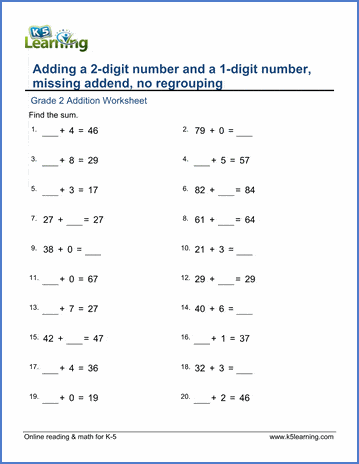Adding within 0-10 4 + 5 =
Add a 2-digit and 1-digit number, no regrouping 34 + 4 =
Add a 2-digit number and a 1-digit number, missing addend 34 + __ = 38
Add two 2-digit numbers, no regrouping 34 + 21 =

Add two 1-digit numbers 6 + 7 =

Add 3 numbers 6 + 4 + 9 =
Add 4 numbers 7 + 8 + 9 + 1 =

## Whole tens

Adding whole tens 30 + 80 =
Adding whole tens, missing number ___ + 80 = 110

Complete the next ten ___ + 8 = 70
Adding by completing whole tens 62+9 = 62+8+1

Add 2-digit and 1-digit numbers 78 + 3 =
Add 2-digit and 1-digit numbers, missing addend 78 + __ = 81

Add a 2-digit number and two 1-digit numbers 87 +2 + 3 =
Add a 2-digit number and three 1-digit numbers 87+2+3+2 =
Add a 2-digit number and three 1-digit numbers - missing addend 87+2+_+2= 94

Add whole tens to a 2-digit number 87 + 40 =
Add a 3-digit number and a 1-digit number 852 + 8 =

Adding whole tens and two 1-digit numbers 20+50+7+9 =
Adding whole tens to a 3-digit number 451 + 30 =
Adding whole tens to a 3-digit number - missing addend 451 +___= 481

## Whole hundreds

Adding whole hundreds to complete a thousand 700+___ =1000

## Adding 2-digit numbers in columns

Add a 2-digit number and a 1-digit number in columns 44
+ 4

Add two 2-digit numbers in columns - no carrying 36
+ 22

Add two 2-digit numbers in columns 67
+ 26

Add three 2-digit numbers in columns 52
+ 47
+ 35

Add four 2-digit numbers in columns 69
+ 97
+ 30
+ 21

Add a 2-digit and a 1-digit number in columns - missing addend 56
+
64

+
50

## Adding 3-digit numbers in columns

Add two 3-digit numbers in columns - no carrying 111
+ 121

Add two 3-digit numbers in columns 397
+ 984

Add three 3-digit numbers in columns 456
+ 678
+ 789

Simple addition word problems (1-2 digits)
Slightly harder addition word problems (1-3 digits)
Mixed addition and subtraction within 20
Mixed addition and subtraction (2 digits)
More mixed addition and subtraction word problems